Smartick is an advanced online program that teaches kids math and coding in only 15 min. a day

Jan11

# Learning How to Subtract Fractions

In this post, we are going to see how to subtract fractions, but for that, you first have to know what the denominator and numerator of a fraction are.

The numerator is the number that is written on top of the fraction and the denominator is the number written on the bottom of the fraction.

To subtract fractions, it is necessary that the fractions have the same denominator. Once the fractions have the same denominator, we just subtract the numerators.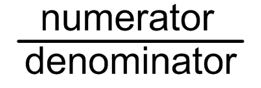What do we do if the fractions have different denominators? In this case, we make the denominators the same by finding their least common multiple (LCM).

Let’s look at an example: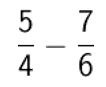Since the denominators are different, 4 and 6, we have to find their least common multiple (LCM). You can review how to calculate the LCM on this previous post on our blog.

## LCM (4,6) = 12

The two new fractions will have 12 as a denominator.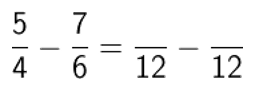In order to find the numerator of each new fraction, divide the new denominator (the LCM that we have found) by the old denominator and multiply the answer by the old numerator.

The first fraction: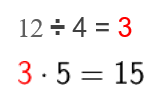The second fraction: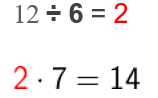So now we have: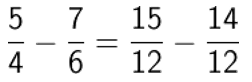As both fractions have the same denominator, now we can subtract the numerators and leave the same denominator: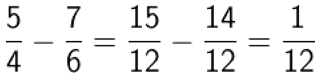And the result is one-twelfth.

If you have liked this post, share it with your friends so that they can also learn how to subtract fractions.